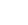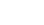# Combinatorics: Definition, Step by Step Articles

Combinatorics is the branch of mathematics that deals with the relations characterizing sets, subsets, lists, and multisets.

Sometimes combinatorics is said to be the branch of math that deals with counting; and that’s true, but not in the sense in which you learned to count in kindergarten. Though combinatorics deals with numbering and finding out how many members are in sets, it’s designed to find ways of doing that without actual, potentially tedious, counting involved.

## Articles

Click on an article name:

## Enumerations, Combinations, Permutations

You’ve probably been introduced to combinatorics when you learned about combinations, permutations, and maybe enumeration.

Enumeration is a way of counting that involves organizing the items to be counted in a complete and systematic list.

Combinations involve, generally speaking, picking a subset out of a larger set. Choosing three students from a class of 26 would be an example of combinations, or picking out three blueberries out of a bush with 600 berries. With combinations, order doesn’t matter.

Permutations are not much different from combinations, but here the set you are picking is an ordered set or list, without repetition. (In combinatorics, we define a list as an ordered sequence of objects). If you’re selecting not just three students, for instance, but a student body president, a vice president, and a secretary, you are picking an ordered list and working with permutations.

Combinatorics also deals with lists that allow repetition; words, made up of the letters of the English language, are a nice example of this. Then there are also multisets, which are non-ordered sets in which repetition is allowed.

## Examples of Combinatorial Problems

A committee of five students have to be chosen out of a student pool of 30. How many possible selections are there?

This classic combinatorics problem is an example of combinations, which uses the combinations formula:Plugging in 30 students total (n), choose five (r) into the formula, we get:
30! / (5! (30 – 5)!) = 30! / (5! * 25!) = 142506.

If each position on the committee was distinguishable, we’d be looking at the number of permutations rather than the number of combinations. This is given by n!/(n-k)!, or 30!/25!, which equals 17100720.

## Combinatorics and Statistics

Combinatorics and statistics are related fields, and statistical research uses many combinatorial methods. In particular, areas like non-parametric statistics, statistical distribution theory, waiting time problems / queuing theory, and the study of urn models are all heavily based on combinatorial problems.

Since combinatorics gives us answers to question about the number of possible outcomes we have when picking subsets from larger sets, combinatorics is also important when designing research projects or studies in social sciences. It forms the groundwork for many probability problems.

## Important Notation in Combinatorics

nk counts the number of lists with k elements taken from an n-element subset. Elements can be repeated, and order matters. This nk is the same as the nk you’re used to working with in algebra: n4 =n*n*n*n
(n)k counts the number of lists with k elements— and no repetition– taken from a set of n elements (think permutations).counts the number of k-element subsets of a set that has n elements. No repetition is allowed, and order doesn’t matter (think combinations).counts the number of k-element multisets that can be taken from a n-element set. Remember, for multisets order doens’t matter, and repetition is allowed.

## References

Grinstead, C. & Snell, J. (2012) Introduction to Probability. American Mathematical Soc.
Guichard, D. (2017). An Introduction to Combinatorics and Graph Theory. Retrieved October 30, 2017 from: https://www.whitman.edu/mathematics/cgt_online/cgt.pdf.
Balakrishnan, N. (1997). Advances in Combinatorial Methods and Applications to Probability and Statistics. Springer Science and Business Media.

CITE THIS AS:
Stephanie Glen. "Combinatorics: Definition, Step by Step Articles" From StatisticsHowTo.com: Elementary Statistics for the rest of us! https://www.statisticshowto.com/probability-and-statistics/combinatorics/
---------------------------------------------------------------------------Need help with a homework or test question? With Chegg Study, you can get step-by-step solutions to your questions from an expert in the field. Your first 30 minutes with a Chegg tutor is free!